# Teaching Binary Using the Vending Machine Example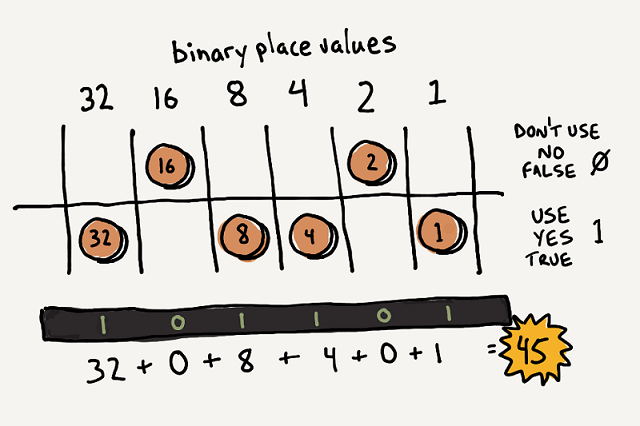DifficultyModerate
Steps
9

In this lesson, students will explore the concept of binary numbers.

Use the example of a vending machine that only accepts Base-2 coins and doesn’t give change!

Students will become familiar with an alternate numbering system, in this case binary (Base-2).

Students will learn how binary relates to decimal, and will be able to convert between the two systems.

Parts Used in This Guide

## Step 1  Preparation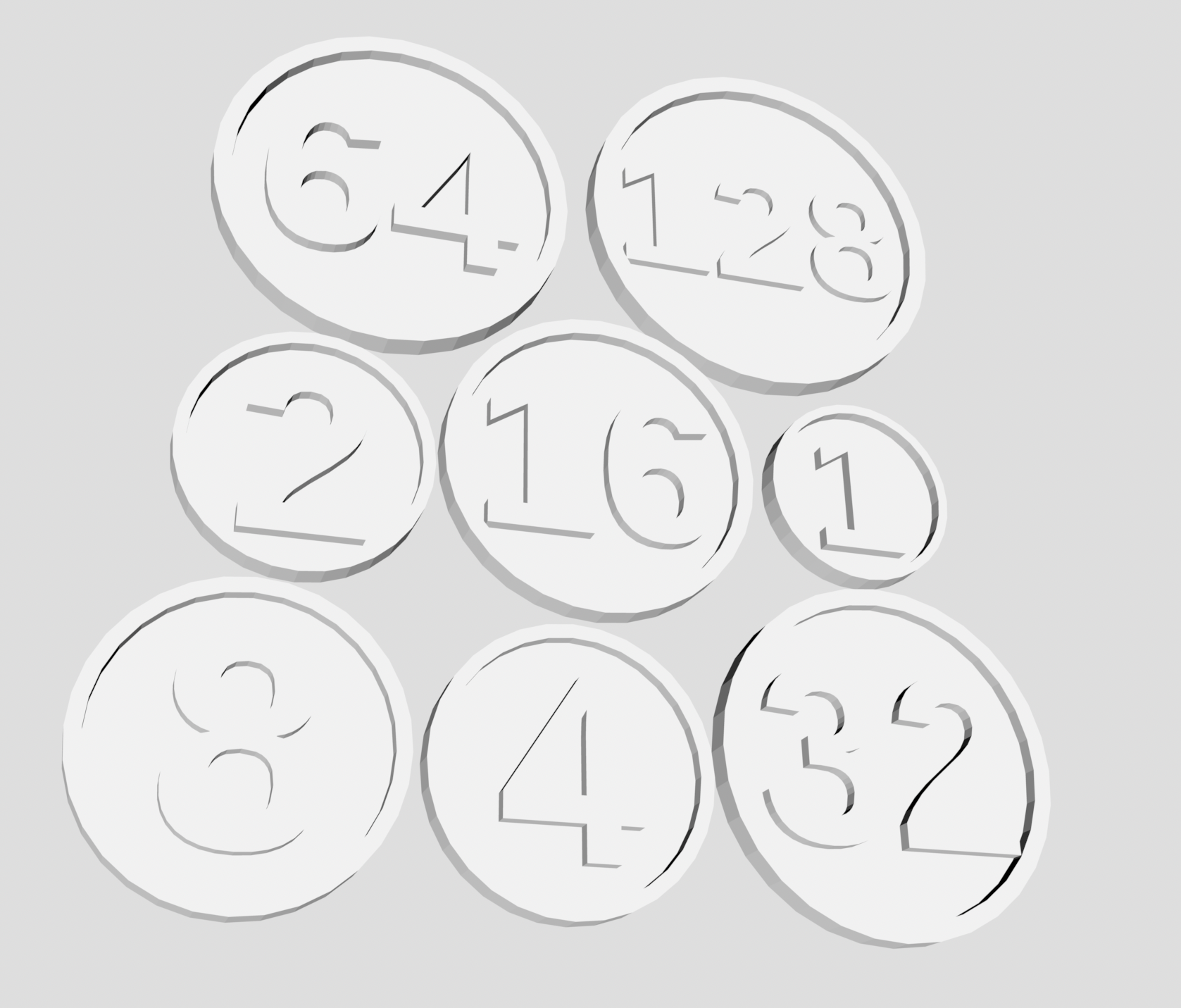Gather or create small counters or ‘coins’ in the following denominations:

• 1, 2, 4, 8, 16, 32.
• Cardboard with the numbers written on each side can be used.
• Make sure to leave one side of each coin blank.

If you have access to a 3D printer you could try these Binary Coins

## Step 2  Introduction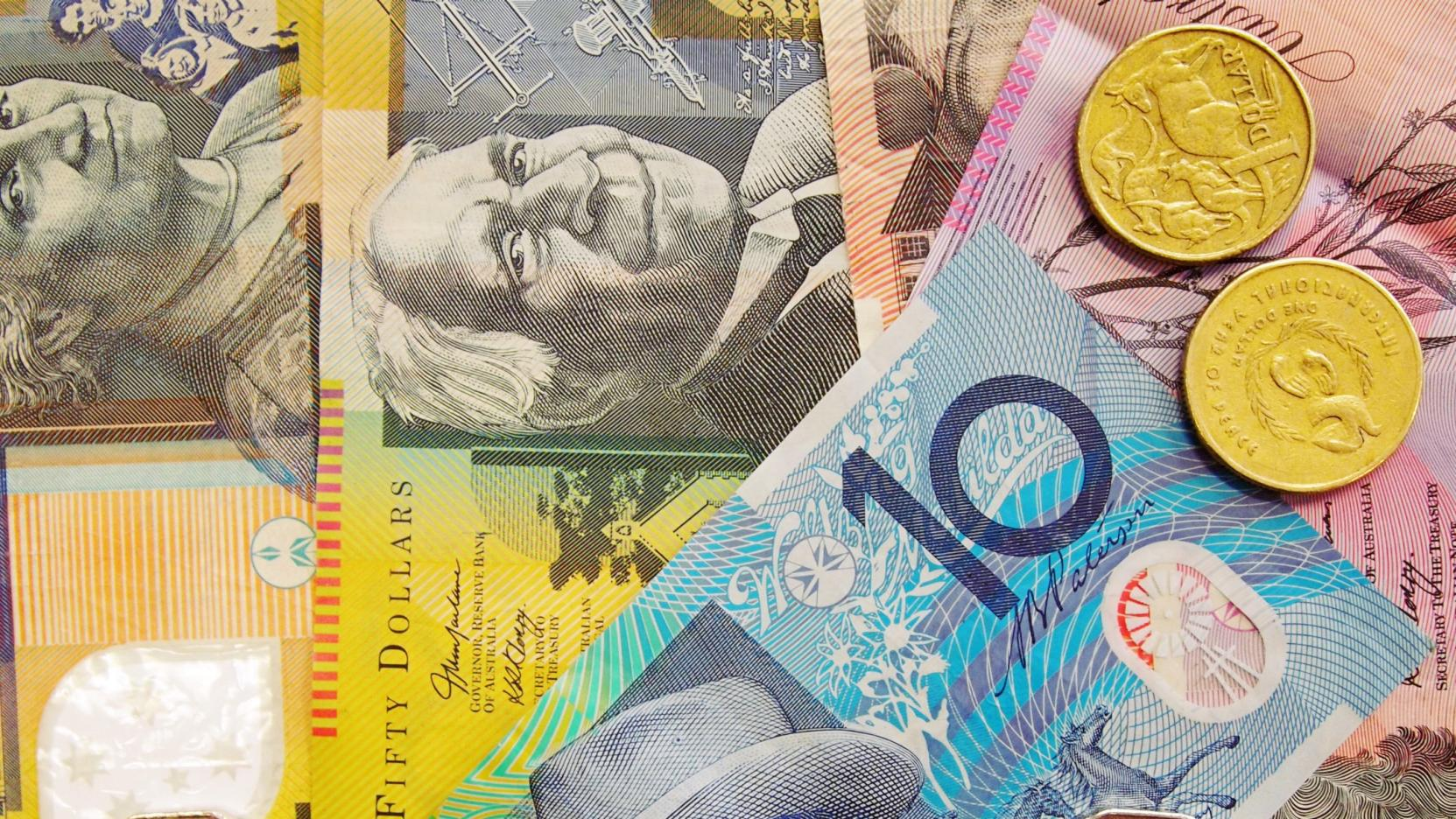Ask the students the following questions to spark discussion:

• Have any of them bought anything in the last 24 hours? Usually they have bought a snack or perhaps lunch.
• Did any of them use cash?
• What bills or coins did they use?
• What are the core denominations of money in Australia?

Lead the students to realise that our core monetary denominations, like our number system are based on ten.

• 1 cent
• 10 cents = 10 single cents
• 1 one dollar coin = 10 ten cents pieces
• 1 ten dollar note = 10 one dollar coins
• 1 hundred dollar note = 10 ten dollar notes

Our money system is based on our number system, the decimal system. The deci- prefix means ‘one tenth’. Each place value in the decimal is one tenth of the place value to its left.

## Step 3  Process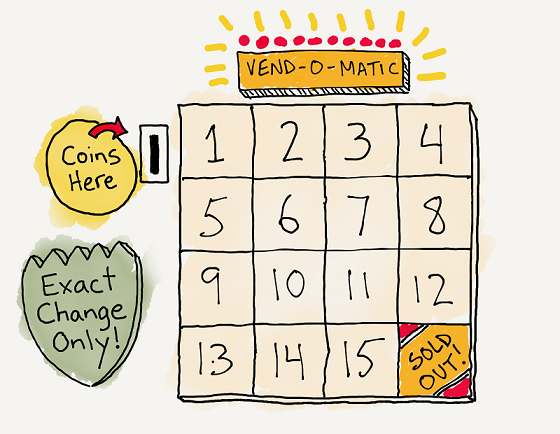Give a set of the coins you prepared earlier to each student or pair of students

Remember to hold onto the 16-unit and 32-unit coins for now

Present the following scenario:

• There is a vending machine that sells items of all prices
• However, the machine cannot give change.
• Therefore, you must pay for everything in exact amounts
• You have one of each coin: 1, 2, 4, 8.

## Step 4  Basic Questions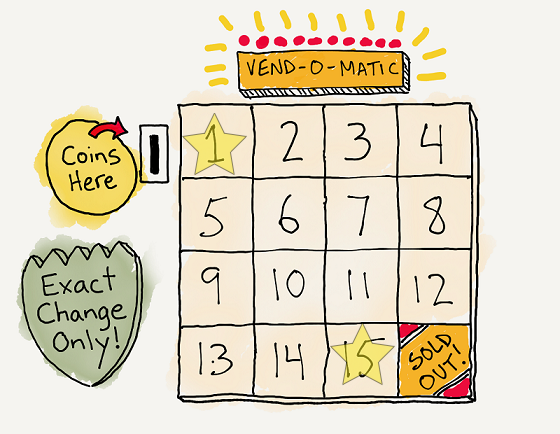What is the price of the least expensive item you can buy? (1 unit) (1)

What is the price of the most expensive item you can buy? (15 units) (1+2+4+8)

What else can you buy? What coin(s) would you use to do this?

What is the price of something you cannot buy, because you don’t have exact change?

Here is where students will start to figure out the different combined sums of different coins. You can also prompt them by saying, for example, “It’s impossible to buy something that costs 11 units, isn’t it?” Someone will immediately point out that you CAN buy an 11-unit item with 8 + 2 + 1.

## Step 5  Observation Questions - Next in sequenceAsk the students to line up the coins in their set from greatest to least denomination, left to right

What do you notice about the denominations as they increase from right to left?

Each amount is double (or times 2 or twice) the denomination before it (to its right).

If we added one more coin to your set of coins that is greater than the 8 unit coin, what is the next logical coin denomination? 16.

Why? Because 16 is ‘2 times’ greater than 8. Hand out the 16 unit coins, one to each student.

## Step 6  New Maximum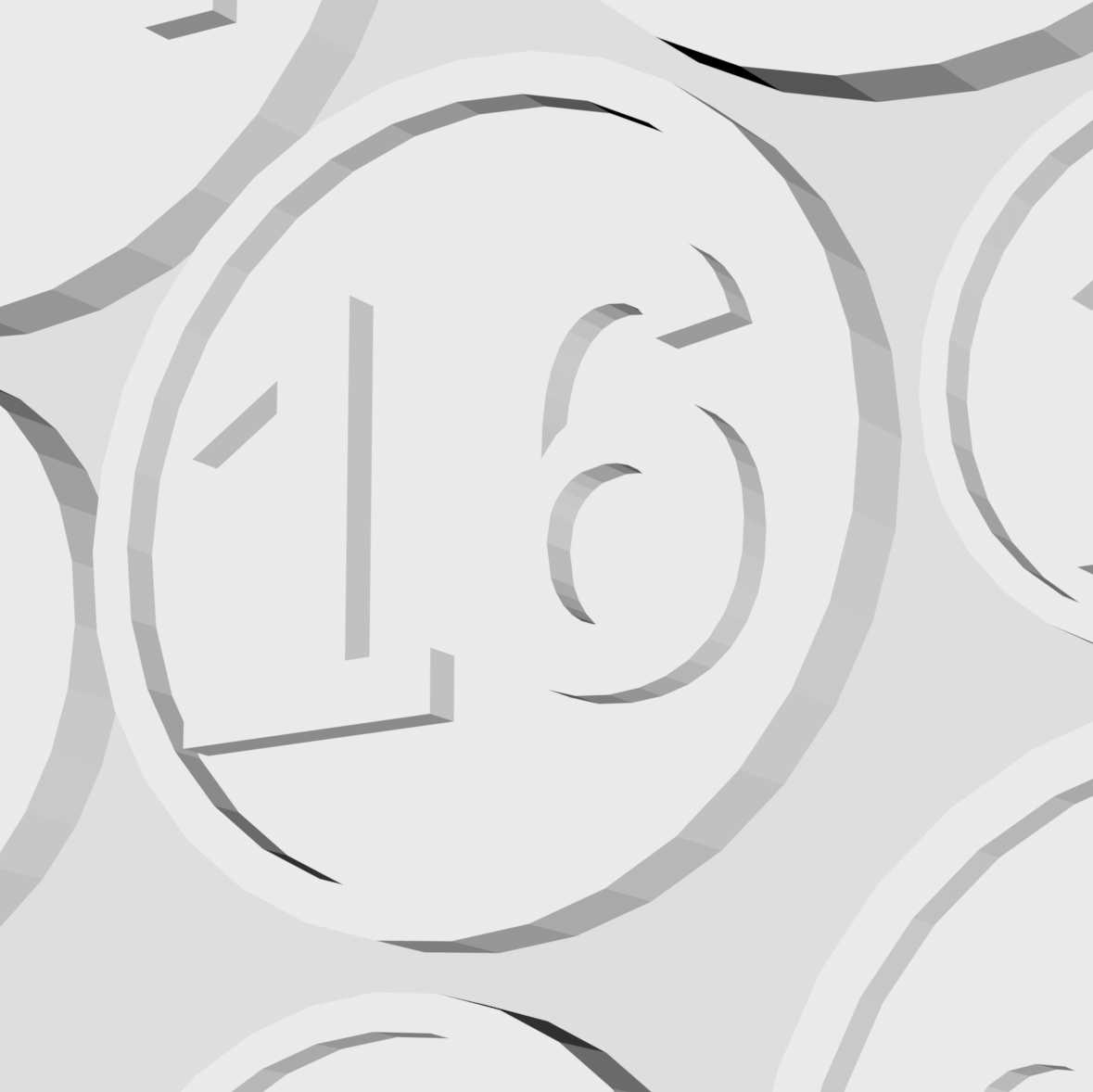What is the new maximum price you could pay for an item? (31 coins) 1+2+4+8+16

What combinations of coins can you use to pay for an item priced from 16 units to this new maximum price?

What is the next coin in the sequence?  Hand out the 32 unit coins, one to each student.

What is the new maximum price you could pay for an item? (63)

What combinations of coins can you use to pay for an item priced from 32 units to this new maximum price?

## Step 7  From coins to binary notationOnce students are comfortable making combinations of numbers, encourage them to use ones and zeroes to represent the numbers instead.

This number system uses the number 2 as its base (each place is two times the one before it.)

It is called the Base-2 system, or binary system. The number system we are normally familiar with is the Base-10 system, or decimal system (each place is ten times the one before it.)

With their coins in a line in descending order on a piece of paper, ask students to represent a given number by keeping face up the coins they would use to make this amount and flipping over or putting face down the coins not used.

Ask them to represent the number 45. See image above.

They should have the 32, 8, 4, and 1 coins face up and the 16 and 2 coins face down. Ask the students to place a numeral 1 above the coins that are face up and a numeral zero over the coins that are face down.

The ones and zeros they just drew are the binary number version of the amount represented by the flipped-up coins. For the example: 45 in Base-10 = 101101 in Base-2

## Step 8  ExamplesPractice Base 2 notation

Examples:

• 22 (1 0 1 1 0 )
• 37 (1 0 0 1 0 1 )

## Step 9  Examples going from Base 2 to Base 10Next, have the students use the above method in reverse to translate numbers from Base-2 to Base-10.

Start with all the coins face up in a line from greatest to least denomination from left to right.

Write the ones and zeros representing the binary number being translated above the coins.

Flip to face down any coin with a zero above it.

Add up the remaining face up coins.

Examples:

• 0 1 0 1 0 (10 )
• 1 1 0 1 1 0 (54 )